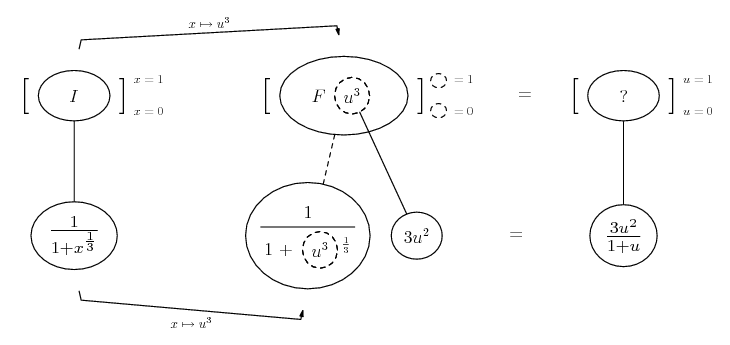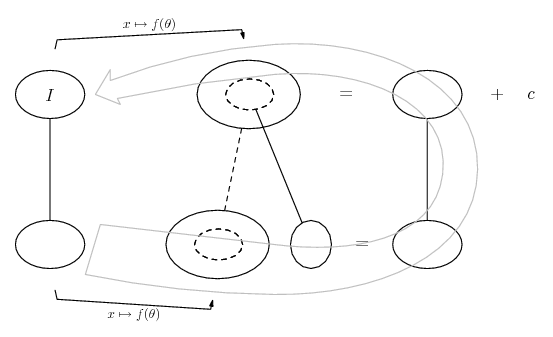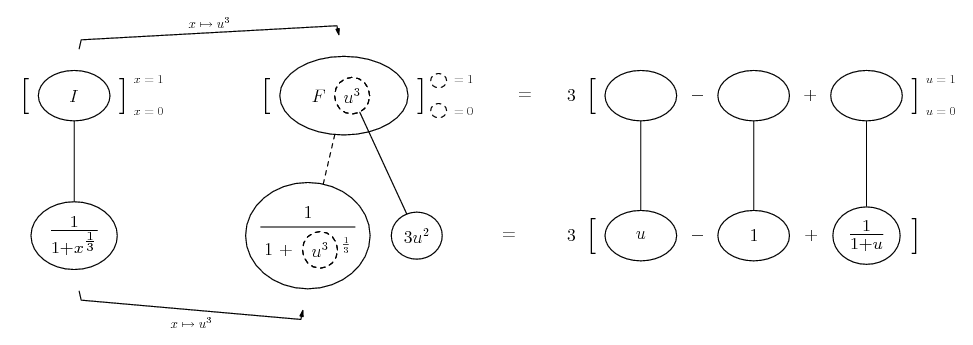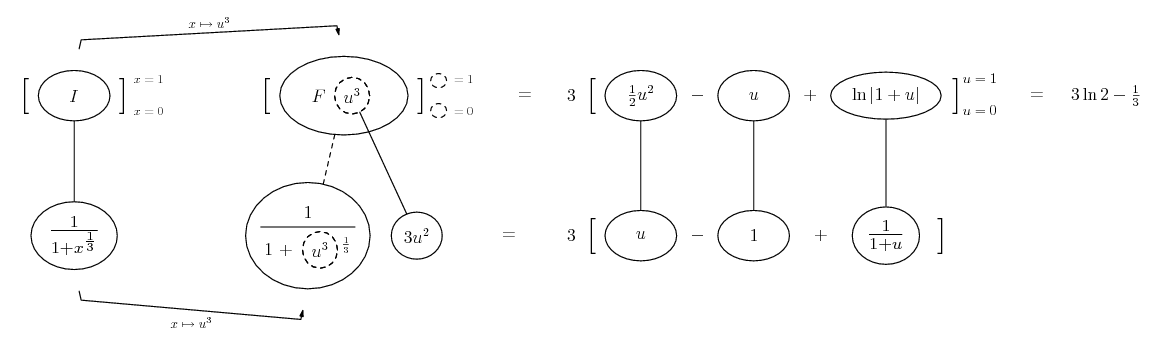# Thread: Evaluating integrals

1. ## Evaluating integrals

Hello! I have done all of my math homework but I'm so stuck on these couple problems.'

0∫1 [ 1/(1+∛x) ]dx (integral between 0 and 1)

and

∫ [ x²/√(1-x²) ]dx

Thank you!!

2. For the second

$\displaystyle \int{\frac{x^2}{\sqrt{1-x^2}}\,dx}$

make the substitution $\displaystyle x = \sin{\theta}$ so that $\displaystyle dx = \cos{\theta}\,d\theta$.

Then the integral becomes

$\displaystyle \int{\frac{\sin^2{\theta}}{\sqrt{1 - \sin^2{\theta}}}\,\cos{\theta}\,d\theta}$

$\displaystyle = \int{\frac{\cos{\theta}\sin^2{\theta}}{\sqrt{\cos^ 2{\theta}}}\,d\theta}$

$\displaystyle = \int{\frac{\cos{\theta}\sin^2{\theta}}{\cos{\theta }}\,d\theta}$

$\displaystyle = \int{\sin^2{\theta}\,d\theta}$

$\displaystyle = \int{\frac{1}{2} - \frac{1}{2}\cos{2\theta}\,d\theta}$

$\displaystyle = \frac{1}{2}\theta - \frac{1}{4}\sin{2\theta} + C$.

Now remembering that $\displaystyle x = \sin{\theta}$ that means $\displaystyle \theta = \arcsin{x}$ and $\displaystyle \cos{\theta} = \sqrt{1 - \sin^2{\theta}} = \sqrt{1 - x^2}$.

So

$\displaystyle \frac{1}{2}\theta - \frac{1}{4}\sin{2\theta} + C = \frac{1}{2}\theta - \frac{1}{2}\sin{\theta}\cos{\theta} + C$

$\displaystyle = \frac{1}{2}\arcsin{x} - \frac{1}{2}x\sqrt{1 - x^2} + C$.

3. Thank you!

4. Just in case a picture helps with the other...... where... (key in spoiler)

Spoiler:... is the chain rule. Straight continuous lines differentiate downwards (integrate up) with respect to x, and the straight dashed line similarly but with respect to the dashed balloon expression (the inner function of the composite which is subject to the chain rule).

The general drift is...Do long division to split up the improper fraction,

$\displaystyle \displaystyle{\frac{u^2}{1 + u}\ =\ \frac{u(1 + u) - (1 + u) + 1}{1 + u}}$

Spoiler:Spoiler:If the new format is shrinking pics, full-size here.

________________________________________

Don't integrate - balloontegrate!

Balloon Calculus; standard integrals, derivatives and methods

Balloon Calculus Drawing with LaTeX and Asymptote!

5.Originally Posted by tom@ballooncalculusJust in case a picture helps with the other...... where... (key in spoiler)

Spoiler:... is the chain rule. Straight continuous lines differentiate downwards (integrate up) with respect to x, and the straight dashed line similarly but with respect to the dashed balloon expression (the inner function of the composite which is subject to the chain rule).

The general drift is...Do long division to split up the improper fraction,

$\displaystyle \displaystyle{\frac{u^2}{1 + u}\ =\ \frac{u(1 + u) - (1 + u) + 1}{1 + u}}$

Spoiler:Spoiler:If the new format is shrinking pics, full-size here.

________________________________________

Don't integrate - balloontegrate!

Balloon Calculus; standard integrals, derivatives and methods

Balloon Calculus Drawing with LaTeX and Asymptote!

Thank you for this. I have never seen this kind of diagram before

6. Or without a picture...

$\displaystyle \int{\frac{1}{1 + \sqrt{x}}\,dx}$.

Let $\displaystyle u = \sqrt{x}$ so that $\displaystyle x = u^3$ and $\displaystyle dx = 3u^2\,du$.

So the integral becomes

$\displaystyle \int{\left(\frac{1}{1 + u}\right)3u^2\,du}$

$\displaystyle = 3\int{\frac{u^2}{1 + u}\,du}$

$\displaystyle = 3\int{u - 1 + \frac{1}{1 + u}\,du}$

$\displaystyle = 3\left(\frac{1}{2}u^2 - u + \ln{|1+u|} + C\right)$.

Now substitute your terminals, remembering that if $\displaystyle x = 0, u = 0$ and if $\displaystyle x = 1, u = 1$.

#### Search Tags

evaluating, integrals